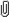September 25, 2023, 04:24:39 am

###AuthorTopic: [Solved] Transformation question  (Read 3220 times) Tweet Share

0 Members and 1 Guest are viewing this topic.

#### jaccs

•• Posts: 7
• Respect: 0##### [Solved] Transformation question
« on: July 28, 2020, 10:10:56 am »
0
Hello, doing a question from the nesa sample paper and getting answer as B, but the solutions are saying it is A. Not sure if i am doing something wrong or the answers are incorrect. any help appreciated
« Last Edit: July 28, 2020, 11:23:23 am by jaccs »

#### fun_jirachi

• MOTM: AUG 18
• Moderator
• Part of the furniture
••• Posts: 1068
• All doom and Gloom.
• Respect: +710##### Re: Transformation question
« Reply #1 on: July 28, 2020, 10:54:42 am »
+3
Hey there, welcome to the forums!Consider what happens when you take each transformation in order.
First, we want to dilate horizontally by a factor of 2 ie. we transform $\sin^{-1}(x-4) \text { to } \sin^{-1}\left(\frac{x-8}{2}\right)$.

Then, we shift the graph right by 1. Note that this actually means we replace $x$ with $x-1$, we don't subtract 1 from the entire expression. The latter is especially problematic as in this case, subtracting 1 from the whole expression actually implies that shifting was done prior to dilation - which is not the case It's important to be careful here, and in any other question that involves this.

Hence, we take the transformation from $\sin^{-1}\left(\frac{x-8}{2}\right) \text{ to } \sin^{-1}\left(\frac{(x-1)-8}{2}\right)$, which gives you the correct answer.

Hope this helpsSpoiler
HSC 2018: Mod Hist  | 2U Maths 
HSC 2019: Physics  | Chemistry  | English Adv  | 3U Maths  | 4U Maths 
ATAR: 99.05

UCAT: 3310 - VR  | DM  | QR  | AR 
Subject Acceleration (2018)
UCAT Question Compilation/FAQ (2020)

#### jaccs

•• Posts: 7
• Respect: 0##### Re: Transformation question
« Reply #2 on: July 28, 2020, 11:22:50 am »
+1
thank you  fun_jirachi
yes, that actually clarifies things enormously and explains why i have been stumbling through these with about 50% accuracy.

i was just dividing the x by 2, not the entire (x - 4) and then after subtracting the 1 was getting and then

thank you so much, that is perfect and so damn obvious now that you have spelled it out in that way!

#### fun_jirachi

• MOTM: AUG 18
• Moderator
• Part of the furniture
••• Posts: 1068
• All doom and Gloom.
• Respect: +710##### Re: [Solved] Transformation question
« Reply #3 on: July 28, 2020, 11:39:14 am »
+3
Yep, it's definitely really important to remember not to apply any direct transformations to constants and only to the variables inside the function. In this example, we're essentially turning $\sin^{-1}(x-4)$ into $\sin^{-1}\left(\frac{x-1}{2}-4\right)$, then rearranging.

Another trick you might want to consider if you're ever in doubt is to sub some easy points in to check you've got things right - this comes especially handy in multiple-choice so you don't have to do any actual computation. An example of a good choice here includes (4, 0) turning into (9, 0) (doubling the distance from the origin and maintaining the sign, then shifting one to the right). A bit more practice will definitely help you push that accuracy up!Spoiler
HSC 2018: Mod Hist  | 2U Maths 
HSC 2019: Physics  | Chemistry  | English Adv  | 3U Maths  | 4U Maths 
ATAR: 99.05

UCAT: 3310 - VR  | DM  | QR  | AR